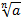Topic Mastery Objectives Essential Knowledge Autumn Term (1st half term) Calculations, checking and rounding · Estimate powers and roots of any given positive number · Apply the product rule for counting Indices, roots, reciprocals and hierarchy of operations · Calculate with fractional and negative indices · Know that a-m = 1/am · Know that am/n = ()m Factors, multiples, primes, standard form and surds · Simplify surd expressions involving squares · Know set notation Algebra: the basics, setting up, rearranging and solving equations- including expanding two brackets containing surds · Simplify and manipulate algebraic expressions (including those involving surds) by expanding products of two binomials and factorising expressions of the form ax2 + bx + c Sequences · Find the nth term of quadratic sequences · Recognise simple geometric progressions Autumn Term (2nd half term) Averages and range · Calculate averages from frequency tables and grouped frequency tables Representing and interpreting data and scatter graphs · Interpolate and extrapolate apparent trends · Know that correlation does not indicate causation · Know the dangers of interpolation and extrapolation Fractions and percentages · Change recurring decimals into their corresponding fractions · Know the corresponding fraction for simple recurring decimals Algebraic Fractions and solving linear equations arising from algebraic fractions · Simplify and manipulate algebraic expressions involving algebraic fractions Ratio and proportion Spring Term (1st half term) Multiplicative reasoning · Set up and solve and interpret answers in growth and decay problems including compound interest Polygons, angles and parallel lines · Know the sum of interior angles of regular polygons · Understand and use the angle properties of parallel lines and find missing angles using the properties of corresponding and alternate angles supplementary, giving reasons · Know the formula to find the sum of interior angles in a polygon · Know the exterior angles in a polygon sum to 360o · Know the angle properties of supplementary, corresponding and alternate angles Pythagoras’ Theorem and trigonometry · Apply Pythagoras theorem in general triangles · Apply the trig ratios to find angles and lengths in right angled triangles, 2D only · Know the convention for labelling the sides in a right-hand triangle · Know the trig ratios · Know the exact values of sinq and cosq, where q = 30, 45, 60, 90 · Know the exact values for tanq, where q = 0, 30, 45, 60 Graphs: the basics and real-life graphs · Draw distance time graphs and velocity time graphs Spring Term (2nd half term) Linear graphs and coordinate geometry · Use the form y = mx + c to identify perpendicular lines · Know that the gradient is calculate using y2 – y1/x2 – x1 · Know the conditions for perpendicular lines Quadratic, cubic and other graphs · Identify roots, intercepts, turning points of quadratic functions graphically Solving quadratic equations and simultaneous equations · Solve quadratic equations (including those requiring rearrangement) algebraically by factorising, by completing the square and using the quadratic formula · Solve two linear simultaneous equations or where one is quadratic, in two variables algebraically · Know the special case of difference of two squares · Know the quadratic formula Solving quadratic equations arising from algebraic fractions Summer Term (1st half term) Inequalities- including quadratic inequalities · Solve linear inequalities in two variables · Solve quadratic inequalities in one variable · Represent the solution set to inequality on a graph Know the conventions for representing inequalities graphically Probability · Calculate and interpret conditional probabilities through representation using expected frequencies with two-way tables, tree diagrams and venn diagrams · Know p(A or B) = p(A) + p(B) · Know p(A and B) = p(A) x p(B) Transformations · Identify describe and construct similar shapes, including on coordinate axes, by considering enlargement (incl. negative scale factor) · Describe changes and invariance achieved by rotations, reflections and translations Know the information required to describe a transformation Summer Term (2nd half term) Similarity and congruence in 2D and 3D Apply concepts of congruence and similarity, including the relationships between length, area and volumes in similar figures Constructions, loci and bearings · Find and describe regions satisfying a combination of loci · Use the standard ruler and compass constructions: · bisect a given angle; · construct a perpendicular to a given line from/at a given point; · construct angles of 90°, 45°; perpendicular bisector of a line segment